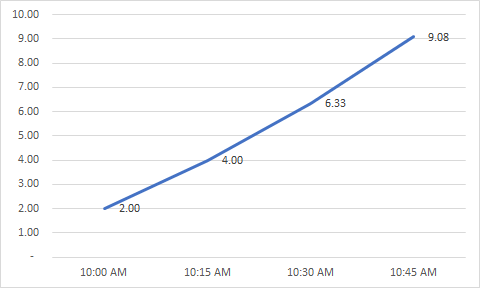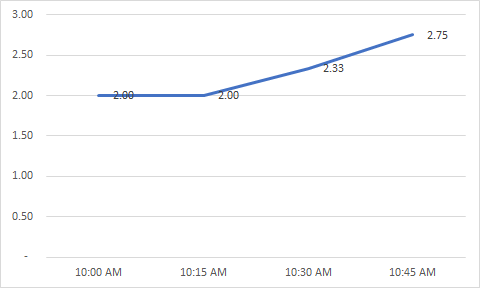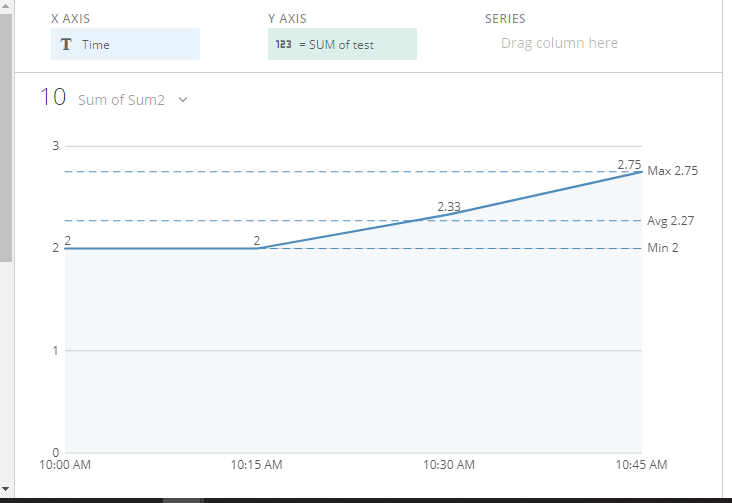# Aggregate Running Total

I am trying to create a running total chart that calculates the aggregate running total as the day goes on.  However, using the running total chart in DOMO, it sums up the amounts per time period rather than take aggregate amounts.  I am hoping someone out there has come up with a solution for the way the chart is built.

To illustrate the problem assume I have a data set that has these amounts:

 Time Amount1 Amount2 10:00 AM 2 1 10:15 AM 2 1 10:30 AM 3 1 10:45 AM 4 1

and I've written a beast mode calc that is Sum(Amount1)/Sum(Amount2).

I put it into a running total chart and DOMO give me this:However, what I want DOMO to do is sum the amounts first, then divide so that I get a chart that looks like this:Has anyone come across this problem before and possibly have a solution?

Thanks.

•CoachI'm not sure if it's possible to do what you're wanting in the running total card.

What I would do is use a SQL transform like so to SUM the values ahead of time. Here's a sample I tested using your numbers:

`SELECT a.`Time`, (SELECT SUM(b.`Amount1`) FROM testing as b WHERE b.`Time` <= a.`Time`) AS 'Sum1', (SELECT SUM(b.`Amount2`) FROM testing as b WHERE b.`Time` <= a.`Time`) AS 'Sum2'FROM testing as a`

Just replace the table name with your own.

I was able to use the results from this, create a basic line card with a BeastMode that was just `Sum1`/`Sum2`

That produced the chart you were looking for.Hopefully that will help.

Sincerely,

ValiantSpur